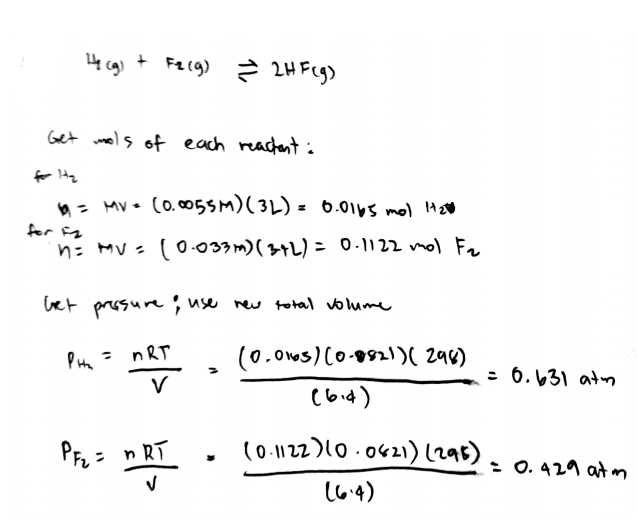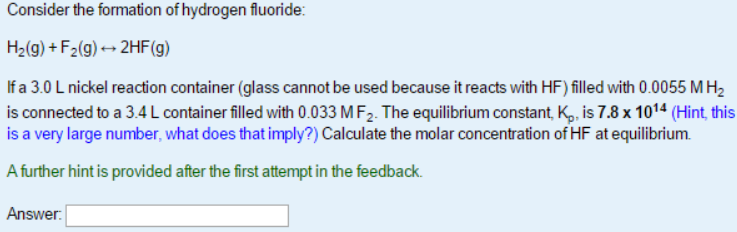# Problem: Consider the formation of hydrogen fluoride: H2 (g) + F2 (g) &lt;-&gt; 2HF (g) If a 3.0 L nickel reaction container (glass cannot be used because it reacts with HF) filled with 0.0055 M H2 is connected to a 3.4 L container filled with 0.033 M F 2. The equilibrium constant. Kp, is 7.8 x 1014. Calculate the molar concentration of HF at equilibrium.

###### FREE Expert Solution81% (330 ratings)###### Problem Details

Consider the formation of hydrogen fluoride:

H2 (g) + F2 (g) <-> 2HF (g)

If a 3.0 L nickel reaction container (glass cannot be used because it reacts with HF) filled with 0.0055 M H2 is connected to a 3.4 L container filled with 0.033 M F 2. The equilibrium constant. Kp, is 7.8 x 1014. Calculate the molar concentration of HF at equilibrium.